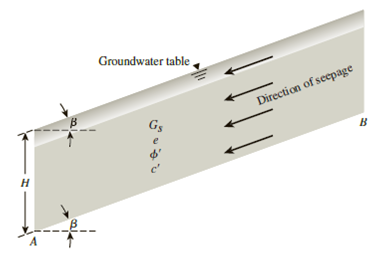Chapter 15, Problem 15.4PPrinciples of Geotechnical Enginee...

9th Edition
Braja M. Das + 1 other
ISBN: 9781305970939

Solutions

Chapter
SectionPrinciples of Geotechnical Enginee...

9th Edition
Braja M. Das + 1 other
ISBN: 9781305970939
Textbook Problem

Figure 15.47 shows an infinite slope with H = 30 ft and the groundwater table coinciding with the ground surface. If there is seepage through the soil, determine the factor of safety against sliding along the plane AB. The soil properties are Gs = 2.7, e = 0.8, β = 16°, ϕ ′ = 21 ° , and c′ = 1250 lb/ft2.Figure 15.47

To determine

Find the factor of safety Fs against sliding along the plane AB.

Explanation

Given information:

The slope rise at an angle β with the horizontal line is 16°.

The height (H) of the soil is 30 ft.

The angle of friction ϕ is 21°.

The cohesion c is 1,250lb/ft2.

The specific gravity Gs of the soil is 2.70.

The void ratio (e) of the soil is 0.8.

Calculation:

Determine the saturated unit weight γsat of the soil using the relation.

γsat=(Gs+e)γw1+e

Here, γw is the unit weight of the water.

Take the unit weight of the water as 62.4lb/ft3.

Substitute 2.70 for Gs, 0.80 for e, and 62.4lb/ft3 for γw.

γsat=(2.70+0.8)(62.4)1+0.8=121.33lb/ft3

Determine the submerged unit weight γ of the soil using the relation.

γ=γsatγw

Substitute 121.33lb/ft3 for γsat and 62.4lb/ft3 for γw

Still sussing out bartleby?

Check out a sample textbook solution.

See a sample solution

The Solution to Your Study Problems

Bartleby provides explanations to thousands of textbook problems written by our experts, many with advanced degrees!

Get Started

What is Hadoop, and what are its basic components?

Database Systems: Design, Implementation, & Management

Define an attribute, and provide three examples.

Systems Analysis and Design (Shelly Cashman Series) (MindTap Course List)

List some applications that use low-pressure piping.

Welding: Principles and Applications (MindTap Course List)